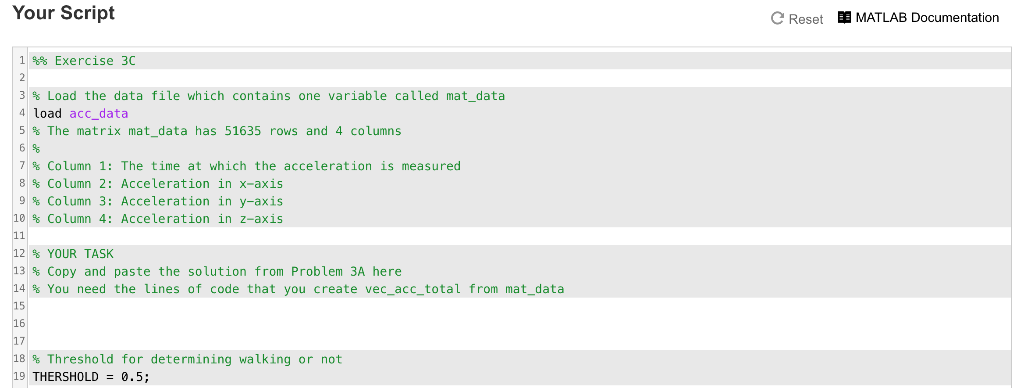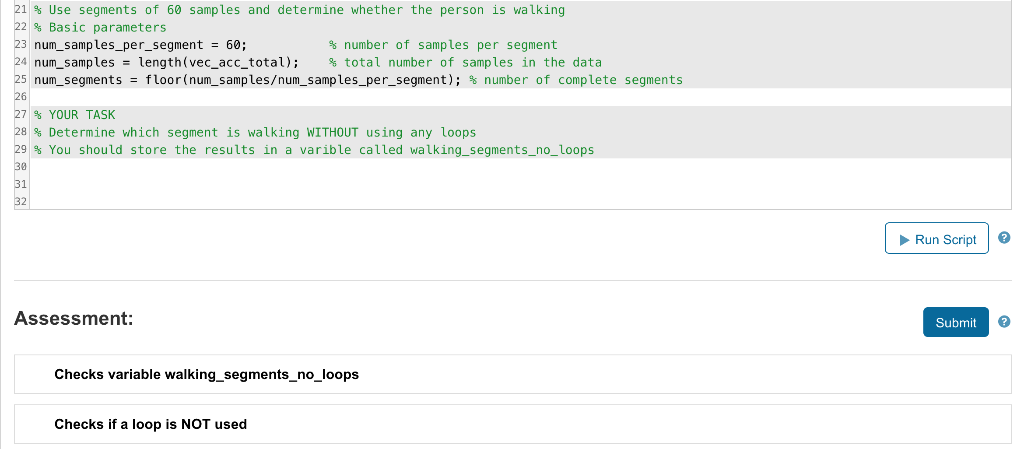# (Solved) : Engg1811 Virtual Lab 2 Matlab Problem 3c Walking Walking Without Using Loops Problem Imple Q37196361 . . .

\$9.00

ENGG1811  Virtual Lab 2: Matlab

Problem 3C: Walking or not walking – WITHOUT using any loops

For this Problem, you should implement the calculations inProblem 3B WITHOUT using any loops.

You will need to use the var() function in Matlab to calculatevariance. You can look at the documentation on how to use thisfunction.

In order to explain what you need to do, let us consider thesmall example in Problem 3B. Let us start from

ex_0 = [2; 20; 2; 10; 10; 10; 10; 9; 10; 3; 18; 3; 6; 7]

which is a vector considering the total accelerations.

You need to extract all the complete segments:

ex_1 = [2; 20; 2; 10; 10; 10; 10; 9; 10; 3; 18; 3]

and then think about using the Matlab reshape() function toobtain the matrix:

2 10 10 3

20 10 9 18

2 10 10 3

Once you get this matrix, you see that each segment is in acolumn. You can then use var() to calculate the variance of thesegments. After that you compare against the threshold.

Note that the Matlab reshape() function stacks columns, you canlook up the examples in the online documentation.

Also if your calculation shows that there are 264 walkingsegments instead of the correct answer of 265 segments, you couldhave used var() in the wrong way. Note that the usage of var() isslightly different from the sum() function that we discuss in thevideo. You should consult the documentation.

You should complete the work in the Your Script box below. Notethat you need to copy and paste your code from Part 3A.

You must store your answer in a column vector calledwalking_segments_no_loops. The test will look for a variable withthat name.

Here is the URL of acc_data.mat:https://www.cse.unsw.edu.au/~en1811/18s2/labs/lab11/acc_data.mat21 % Use segments of 60 samples and determine whether the person is walking 22% Basic pa rameters 3 num_samples_per_segment 60; 24 num-samples- length(vec-acc-total); % total number of samples in the data 25 num-segments-floor(nun_samples/num-samples-per-segment); % number of complete segments 26 27 % YOUR TASK 28 % Determine which segment is walking WITHOUT using any loops 29% You should store the results in a varible called walking-segments-no-loops 30 number of samples per segment 31 Run Script Assessment: Submit Checks variable walking_segments_no_loops Checks if a loop is NOT used

. . .

Category:

## Description

ENGG1811  Virtual Lab 2: Matlab

Problem 3C: Walking or not walking – WITHOUT using any loops

For this Problem, you should implement the calculations inProblem 3B WITHOUT using any loops.

You will need to use the var() function in Matlab to calculatevariance. You can look at the documentation on how to use thisfunction.

In order to explain what you need to do, let us consider thesmall example in Problem 3B. Let us start from

ex_0 = [2; 20; 2; 10; 10; 10; 10; 9; 10; 3; 18; 3; 6; 7]

which is a vector considering the total accelerations.

You need to extract ... View full description